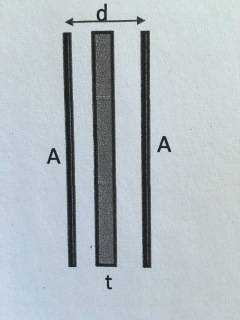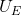# Parallel plate capacitor

## Homework Statement

Consider a parallel plate capacitor of area A and separation d. The plates are isloated. One has charge +Q and the other -Q. An isolated conducting plate of area A and thickness t is inserted between the plates as shown.a. Find the E fields between the conductors

b. Find the potentials between the conductors

c. Using the above, find the capacitance of this new arrangement and compare with C before inserting the conducting plate.

d. Calaculate the energy densityof the charged capacitors before and after inserting the conducting plate

σ = Q / A

## The Attempt at a Solution

a. From Gauss's law, the E field for parallel plates E = σ / ε = Q / Aε , for the plate that is negatively charged, E = - Q / Aε

b. From V(x) = -∫E dx (from 0 to x), The potential is V = E * d = Qd / Aε , but we want to find the potential to the slab in the middle, so substitute d with d/2 + t/2, then V = E * (d+t)/2 = (Q(d+t)/2) / Aε = Q(d+t) / 2Aε

c. The capacitance C = Q/V
C = Q / (Q(d+t) / 2Aε) = 2Aε / (d+t)
Without the slab in the middle, C = 2Aε / d

I hope my steps are correct so far, and I don't know how to find the energy density here, I believe the energy density of a field is 1/2 * ε E^2 , but not sure how the slab would affect it